# Frank Solutions for Class 10 Maths Chapter 19 Mensuration I

Frank Solutions for Class 10 Maths Chapter 19 Mensuration I are provided here. The solutions given on this page contain detailed step-by-step explanations of all the problems in this chapter. These solutions will help you in gaining knowledge and strong command over the subject. The solutions, prepared by our expert faculty team, help students in their exam preparation to secure full marks in Maths. If you wish to secure an excellent score, solving Frank Solutions for Class 10 Maths Chapter 19 is a must.

Chapter 19 – Mensuration I contains topics related to the circumference of a circle and the area of a circle. We, at BYJU’S, have prepared the solutions with detailed descriptions. Students can score full marks in the Maths exam by practising Frank Solutions for Class 10 Maths.

## Download the PDF of Frank Solutions for Class 10 Maths Chapter 19 Mensuration I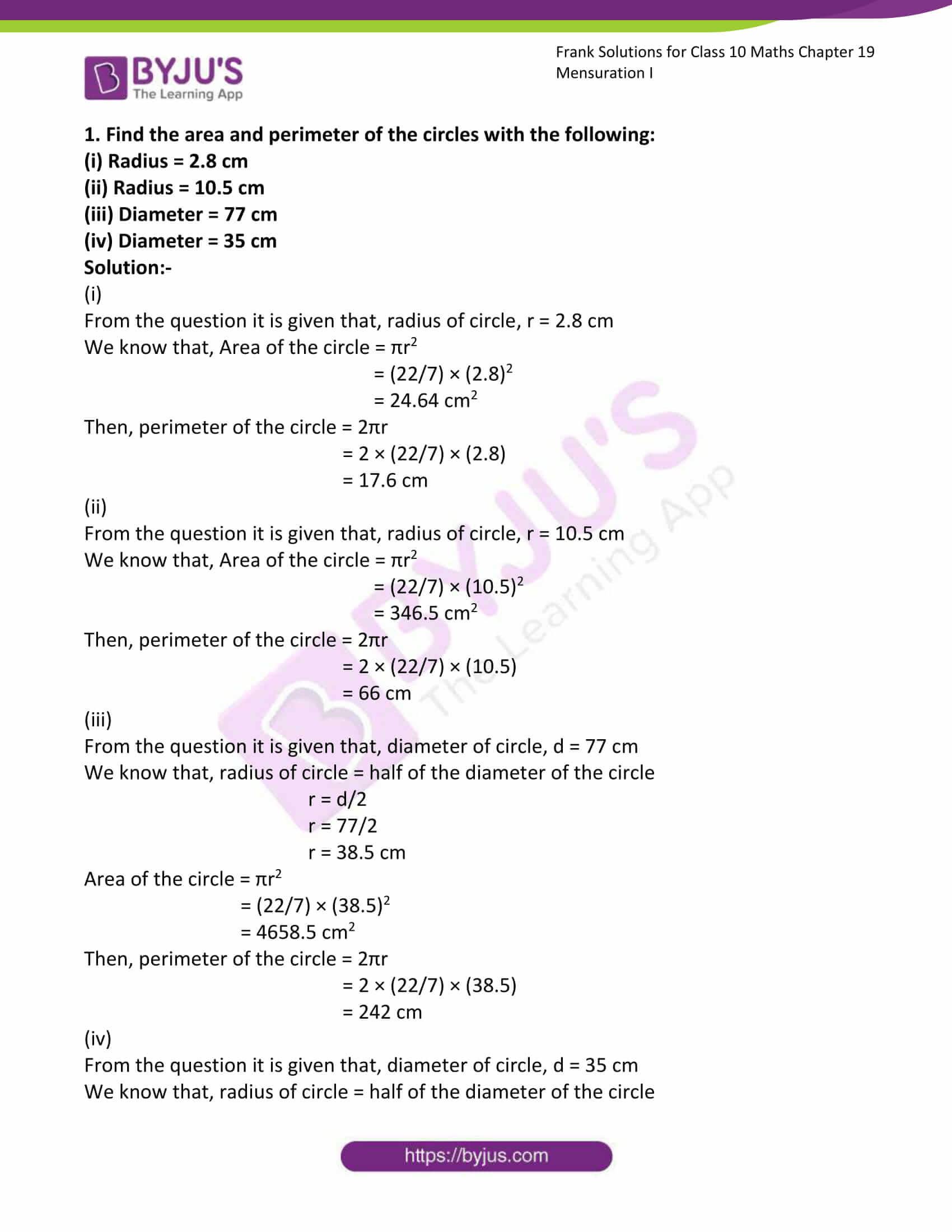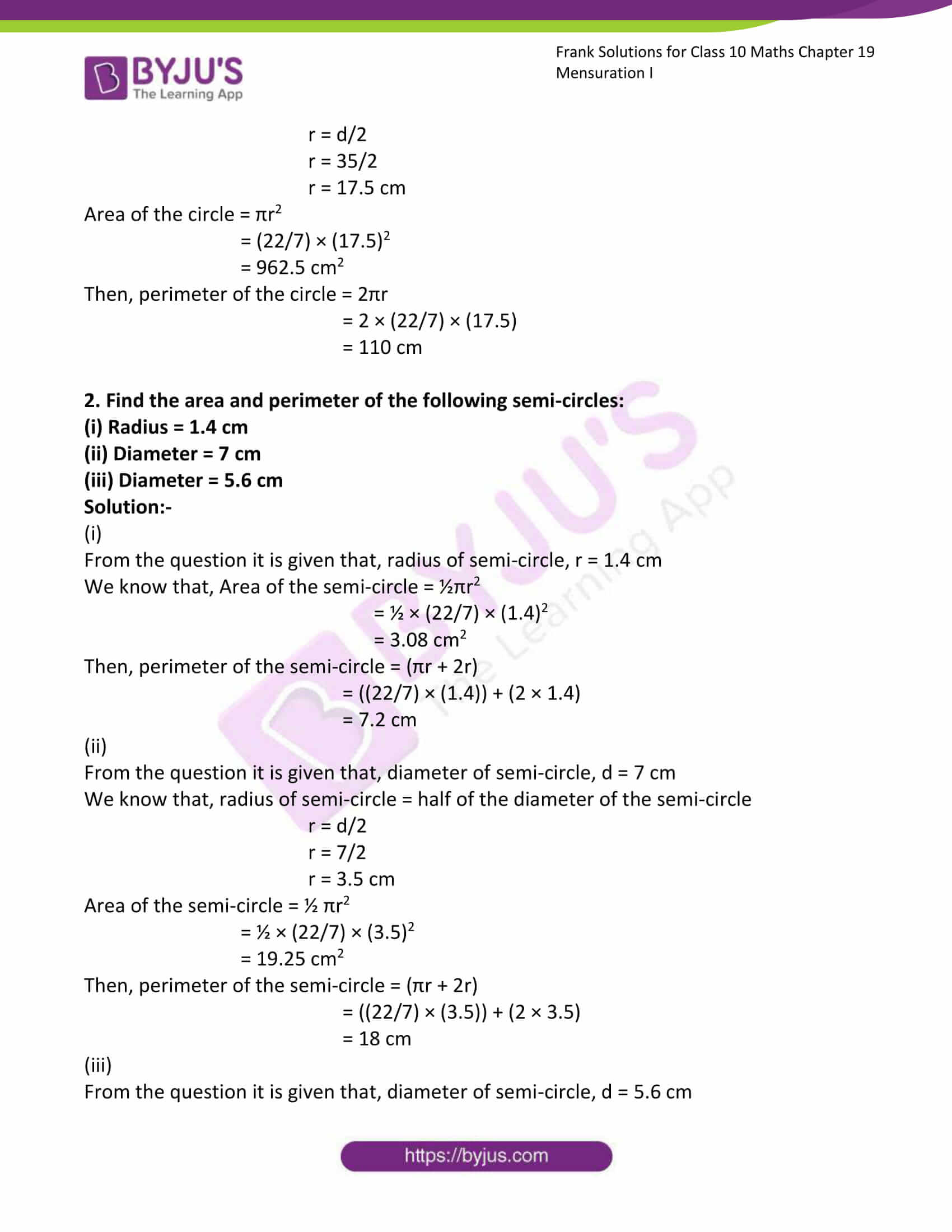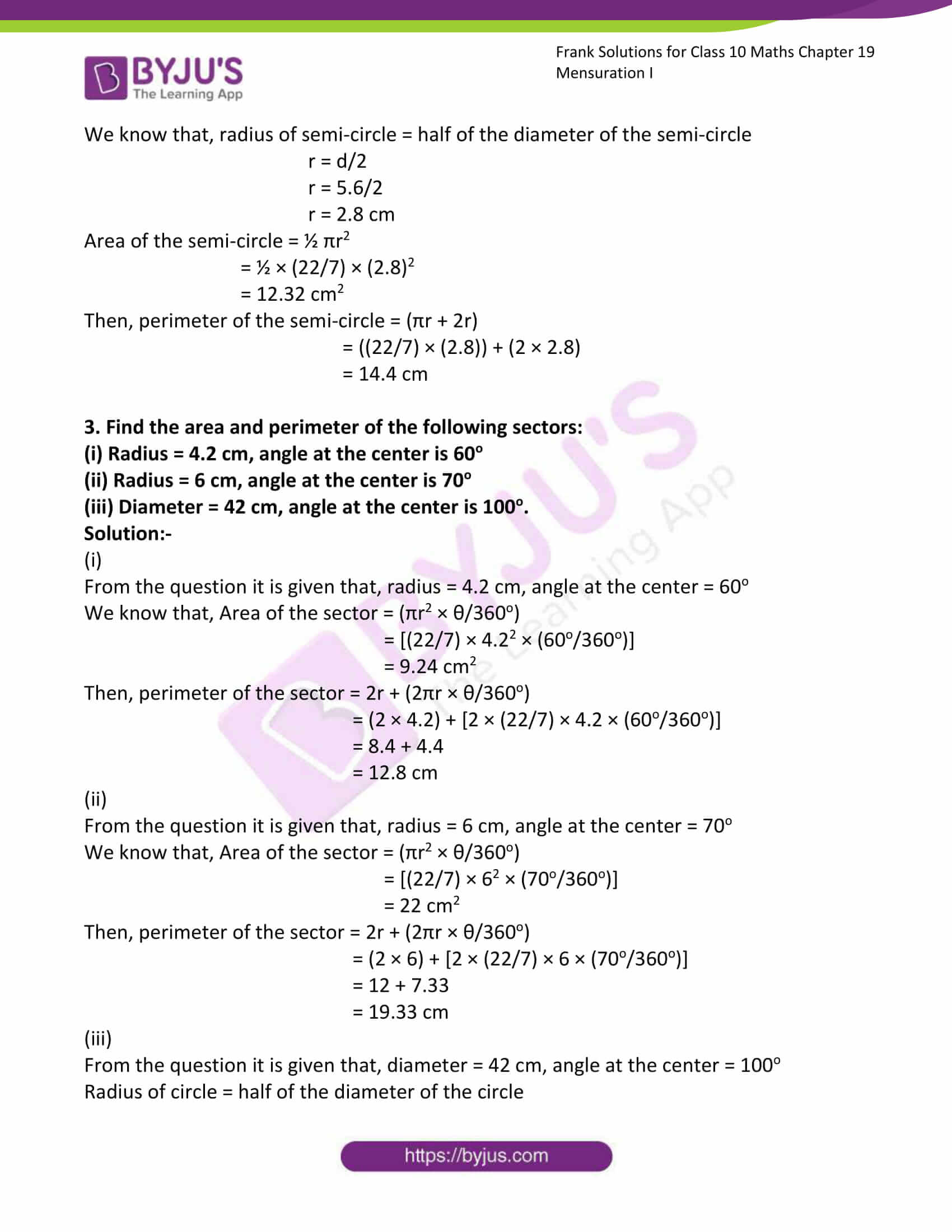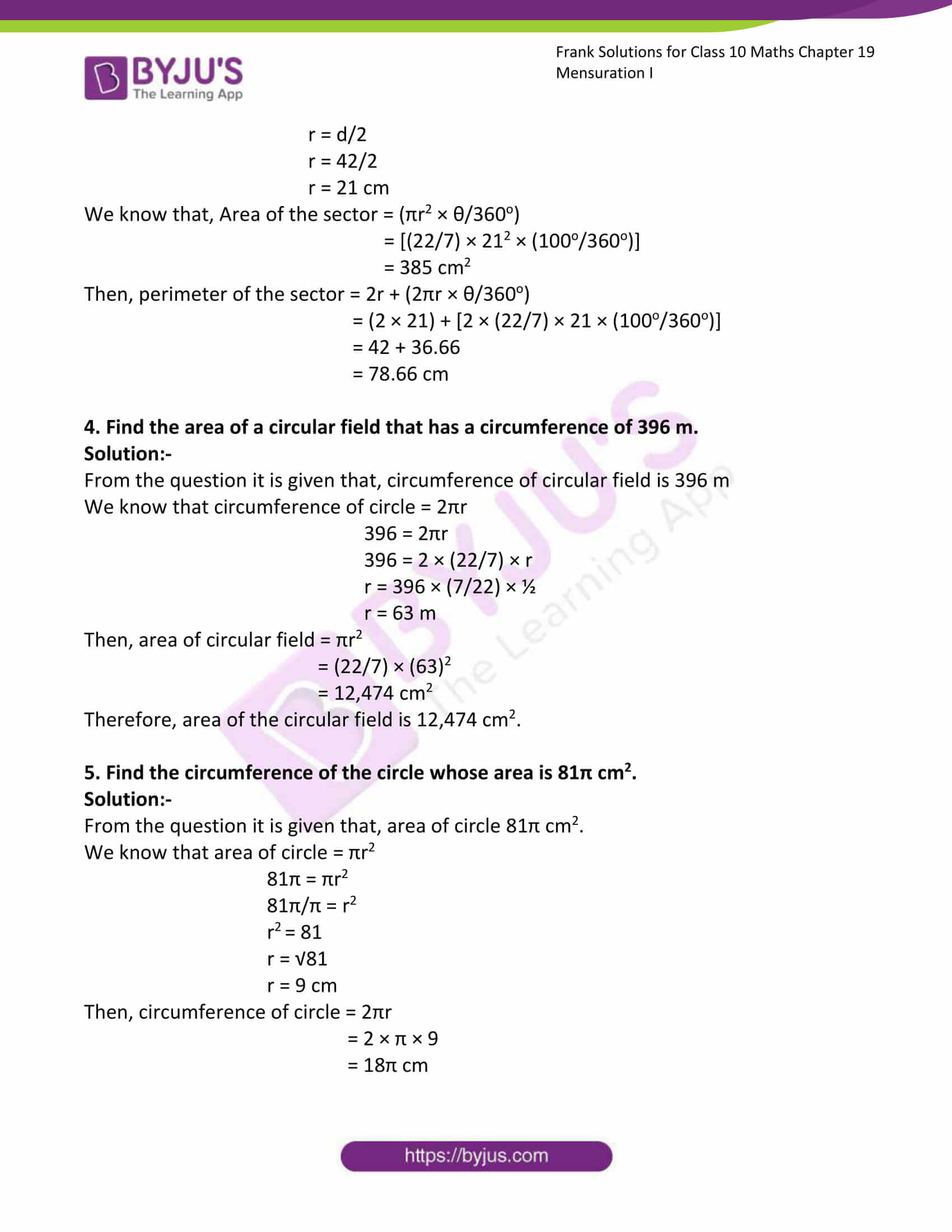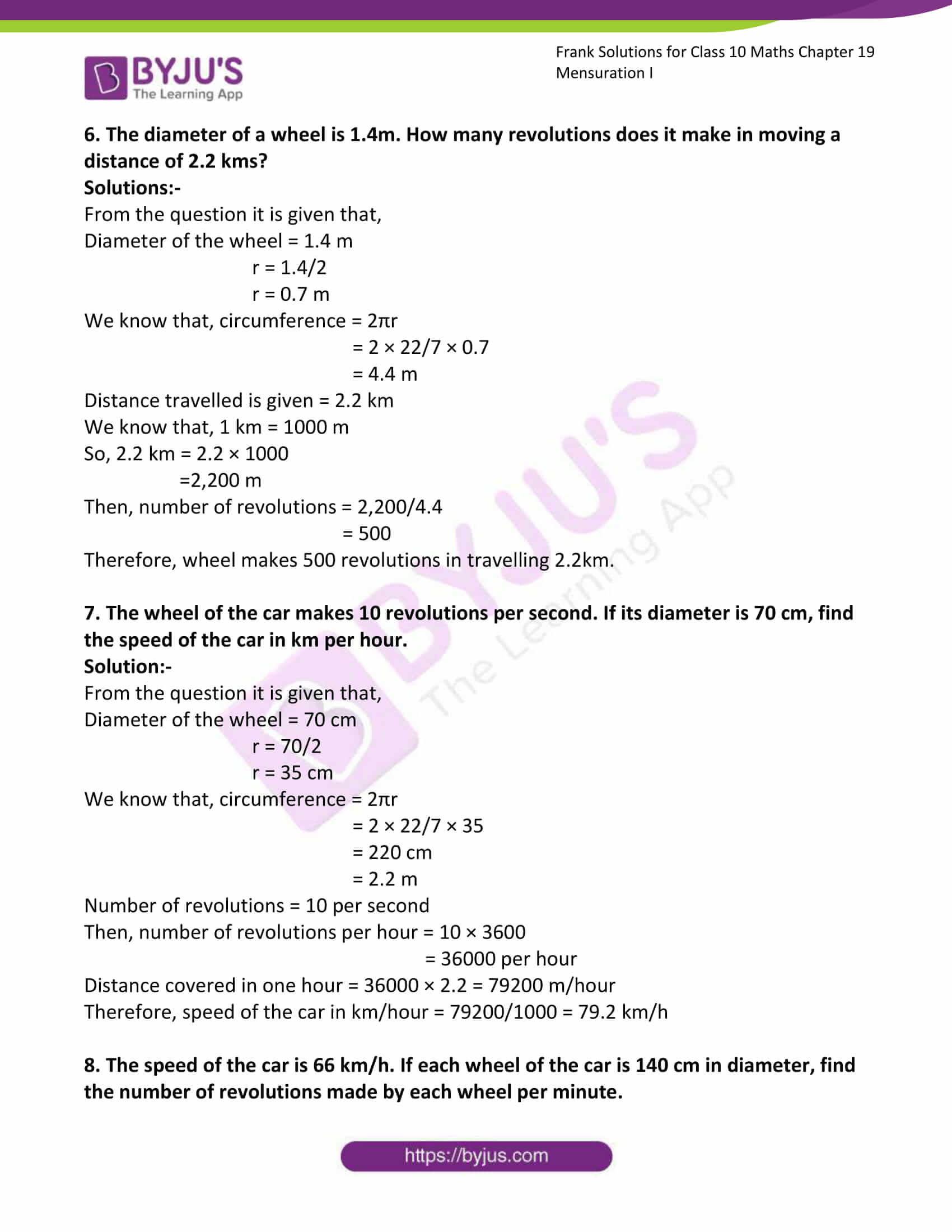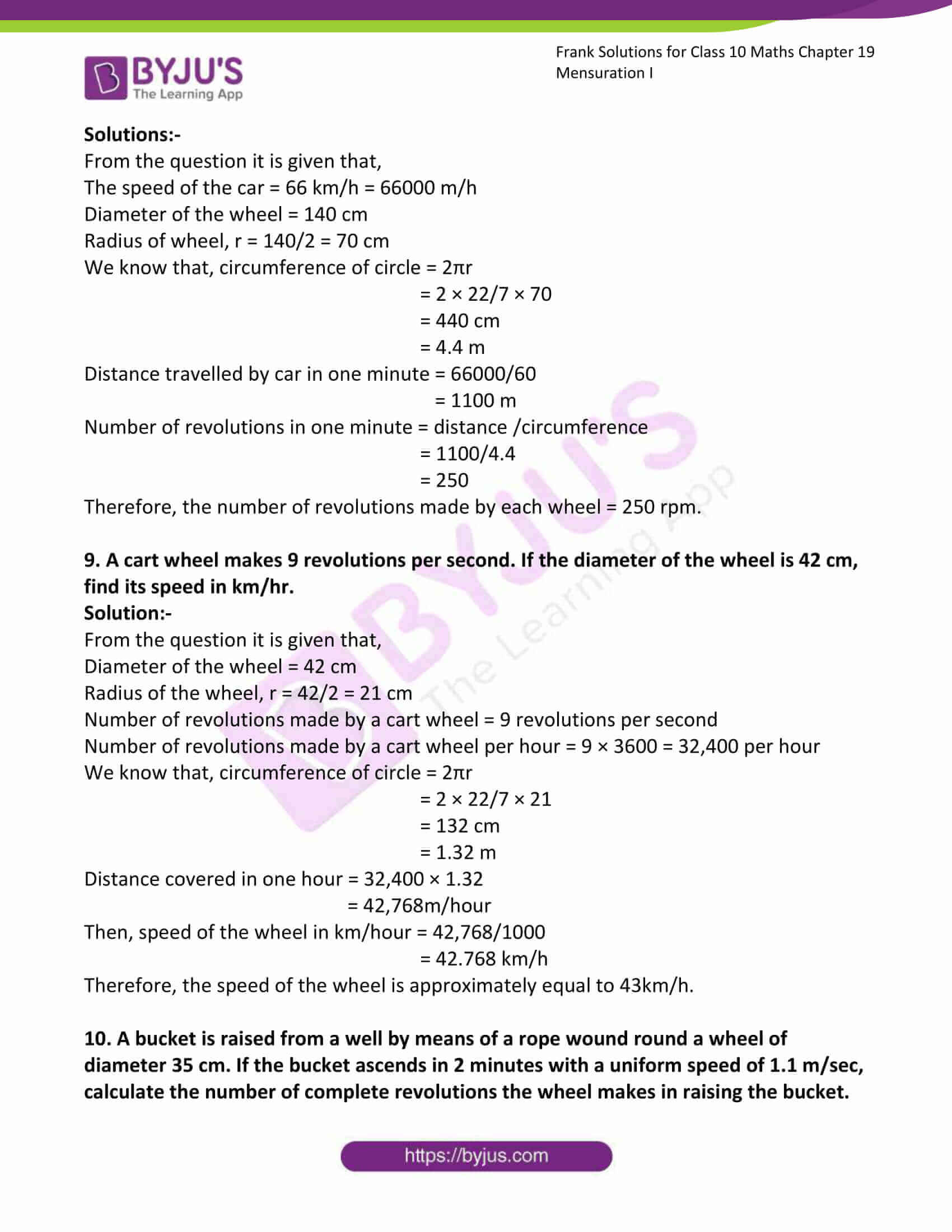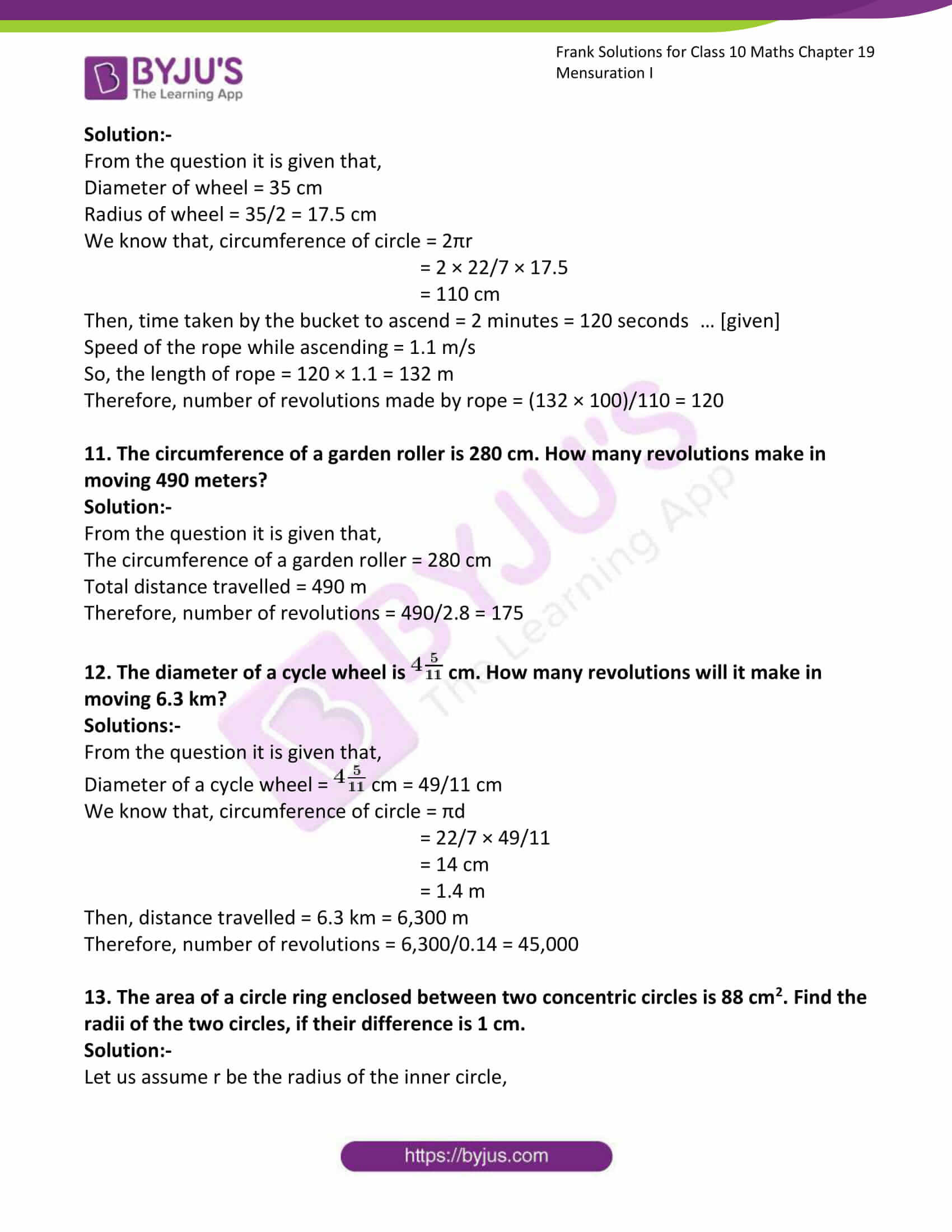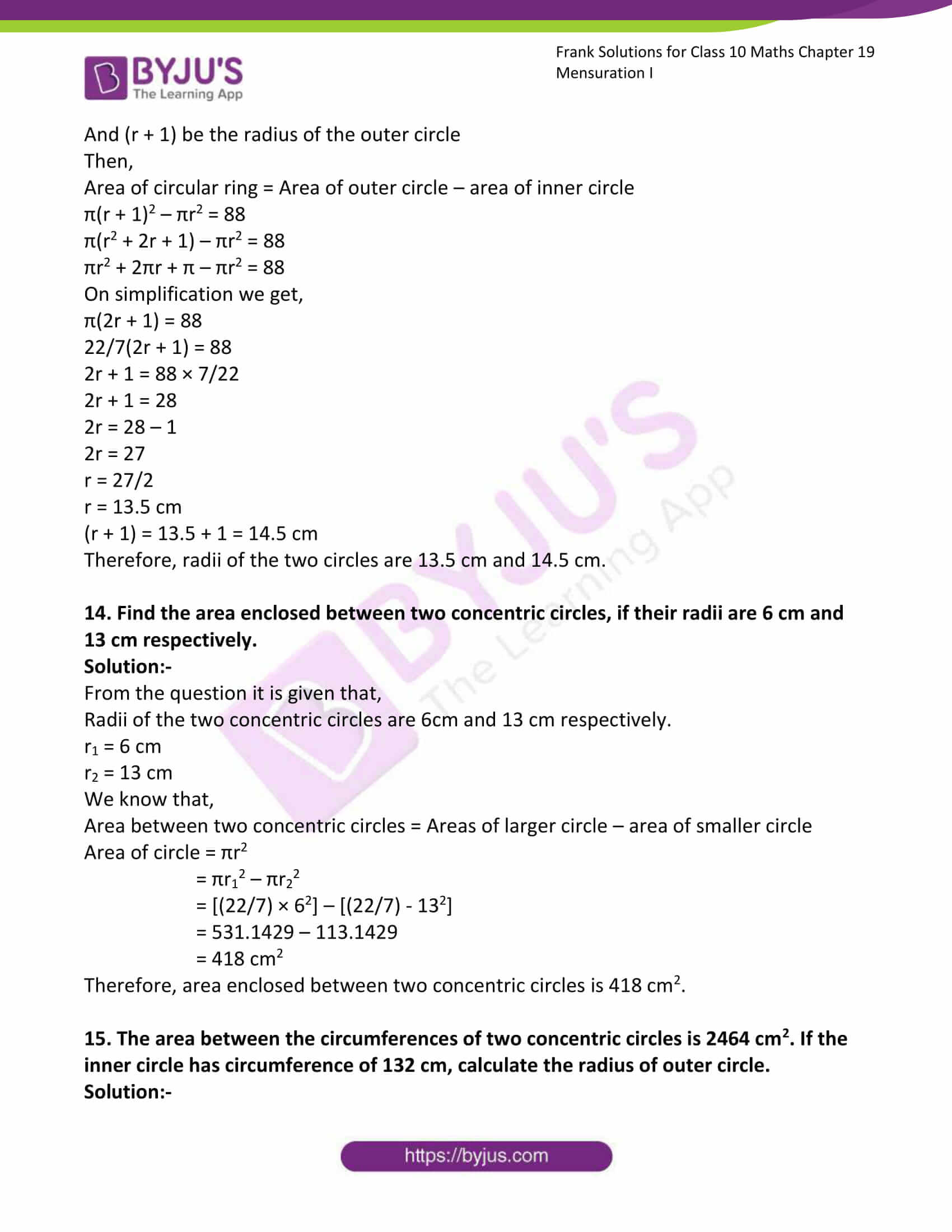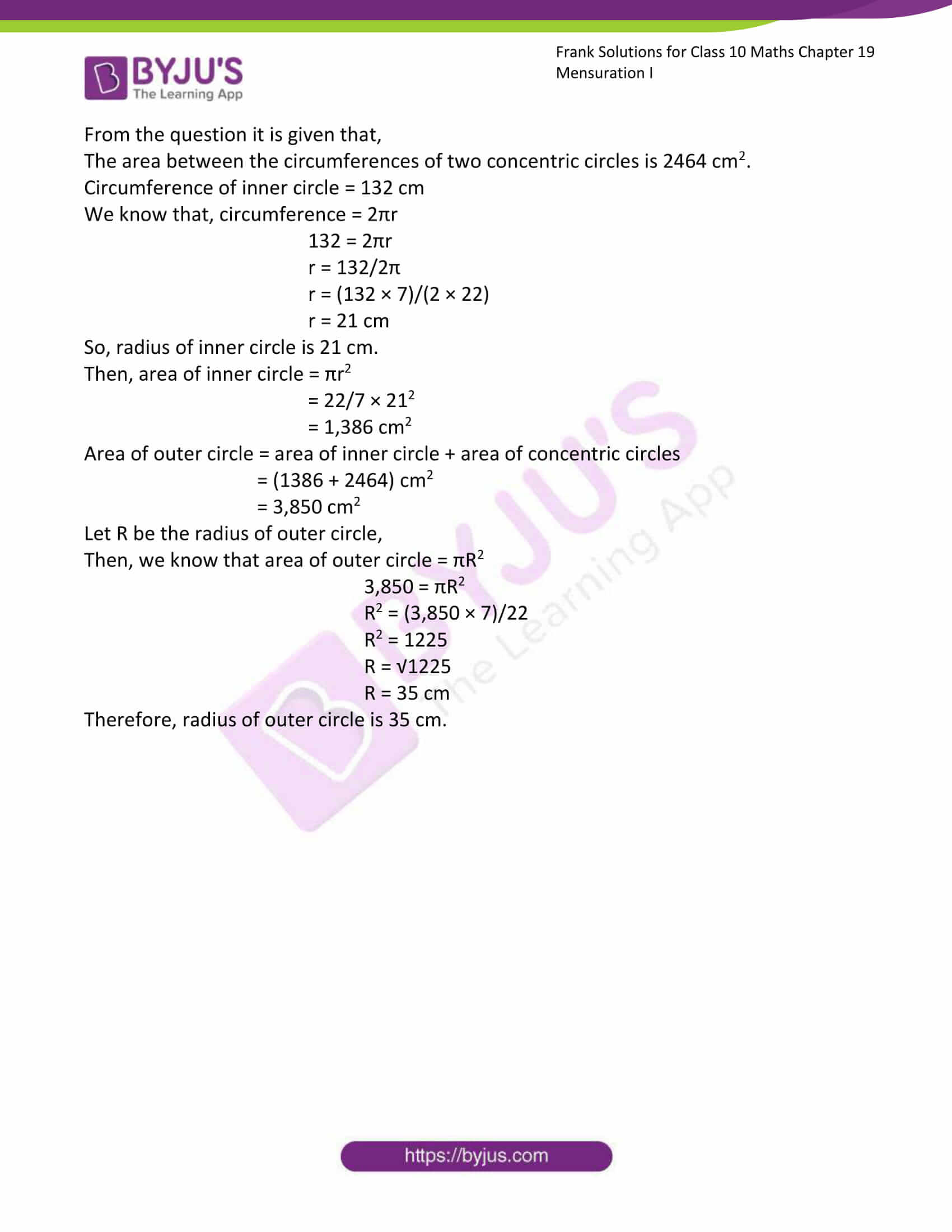### Access Answers to Frank Solutions for Class 10 Maths Chapter 19 Mensuration I

1. Find the area and perimeter of the circles with the following:

(iii) Diameter = 77 cm

(iv) Diameter = 35 cm

Solution:-

(i)

From the question it is given that, radius of circle, r = 2.8 cm

We know that, Area of the circle = πr2

= (22/7) × (2.8)2

= 24.64 cm2

Then, perimeter of the circle = 2πr

= 2 × (22/7) × (2.8)

= 17.6 cm

(ii)

From the question it is given that, radius of circle, r = 10.5 cm

We know that, Area of the circle = πr2

= (22/7) × (10.5)2

= 346.5 cm2

Then, perimeter of the circle = 2πr

= 2 × (22/7) × (10.5)

= 66 cm

(iii)

From the question it is given that, diameter of circle, d = 77 cm

We know that, radius of circle = half of the diameter of the circle

r = d/2

r = 77/2

r = 38.5 cm

Area of the circle = πr2

= (22/7) × (38.5)2

= 4658.5 cm2

Then, perimeter of the circle = 2πr

= 2 × (22/7) × (38.5)

= 242 cm

(iv)

From the question it is given that, diameter of circle, d = 35 cm

We know that, radius of circle = half of the diameter of the circle

r = d/2

r = 35/2

r = 17.5 cm

Area of the circle = πr2

= (22/7) × (17.5)2

= 962.5 cm2

Then, perimeter of the circle = 2πr

= 2 × (22/7) × (17.5)

= 110 cm

2. Find the area and perimeter of the following semi-circles:

(ii) Diameter = 7 cm

(iii) Diameter = 5.6 cm

Solution:-

(i)

From the question it is given that, radius of semi-circle, r = 1.4 cm

We know that, Area of the semi-circle = ½πr2

= ½ × (22/7) × (1.4)2

= 3.08 cm2

Then, perimeter of the semi-circle = (πr + 2r)

= ((22/7) × (1.4)) + (2 × 1.4)

= 7.2 cm

(ii)

From the question it is given that, diameter of semi-circle, d = 7 cm

We know that, radius of semi-circle = half of the diameter of the semi-circle

r = d/2

r = 7/2

r = 3.5 cm

Area of the semi-circle = ½ πr2

= ½ × (22/7) × (3.5)2

= 19.25 cm2

Then, perimeter of the semi-circle = (πr + 2r)

= ((22/7) × (3.5)) + (2 × 3.5)

= 18 cm

(iii)

From the question it is given that, diameter of semi-circle, d = 5.6 cm

We know that, radius of semi-circle = half of the diameter of the semi-circle

r = d/2

r = 5.6/2

r = 2.8 cm

Area of the semi-circle = ½ πr2

= ½ × (22/7) × (2.8)2

= 12.32 cm2

Then, perimeter of the semi-circle = (πr + 2r)

= ((22/7) × (2.8)) + (2 × 2.8)

= 14.4 cm

3. Find the area and perimeter of the following sectors:

(i) Radius = 4.2 cm, angle at the centre is 60o

(ii) Radius = 6 cm, angle at the centre is 70o

(iii) Diameter = 42 cm, angle at the centre is 100o.

Solution:-

(i)

From the question it is given that, radius = 4.2 cm, angle at the centre = 60o

We know that, Area of the sector = (πr2 × θ/360o)

= [(22/7) × 4.22 × (60o/360o)]

= 9.24 cm2

Then, perimeter of the sector = 2r + (2πr × θ/360o)

= (2 × 4.2) + [2 × (22/7) × 4.2 × (60o/360o)]

= 8.4 + 4.4

= 12.8 cm

(ii)

From the question it is given that, radius = 6 cm, angle at the centre = 70o

We know that, Area of the sector = (πr2 × θ/360o)

= [(22/7) × 62 × (70o/360o)]

= 22 cm2

Then, perimeter of the sector = 2r + (2πr × θ/360o)

= (2 × 6) + [2 × (22/7) × 6 × (70o/360o)]

= 12 + 7.33

= 19.33 cm

(iii)

From the question it is given that, diameter = 42 cm, angle at the centre = 100o

Radius of circle = half of the diameter of the circle

r = d/2

r = 42/2

r = 21 cm

We know that, Area of the sector = (πr2 × θ/360o)

= [(22/7) × 212 × (100o/360o)]

= 385 cm2

Then, perimeter of the sector = 2r + (2πr × θ/360o)

= (2 × 21) + [2 × (22/7) × 21 × (100o/360o)]

= 42 + 36.66

= 78.66 cm

4. Find the area of a circular field that has a circumference of 396 m.

Solution:-

From the question it is given that, circumference of circular field is 396 m

We know that circumference of circle = 2πr

396 = 2πr

396 = 2 × (22/7) × r

r = 396 × (7/22) × ½

r = 63 m

Then, area of circular field = πr2

= (22/7) × (63)2

= 12,474 cm2

Therefore, area of the circular field is 12,474 cm2.

5. Find the circumference of the circle whose area is 81π cm2.

Solution:-

From the question it is given that, area of circle 81π cm2.

We know that area of circle = πr2

81π = πr2

81π/π = r2

r2 = 81

r = √81

r = 9 cm

Then, circumference of circle = 2πr

= 2 × π × 9

= 18π cm

6. The diameter of a wheel is 1.4m. How many revolutions does it make in moving a distance of 2.2 kms?

Solutions:-

From the question it is given that,

Diameter of the wheel = 1.4 m

r = 1.4/2

r = 0.7 m

We know that, circumference = 2πr

= 2 × 22/7 × 0.7

= 4.4 m

Distance travelled is given = 2.2 km

We know that, 1 km = 1000 m

So, 2.2 km = 2.2 × 1000

=2,200 m

Then, number of revolutions = 2,200/4.4

= 500

Therefore, wheel makes 500 revolutions in travelling 2.2km.

7. The wheel of the car makes 10 revolutions per second. If its diameter is 70 cm, find the speed of the car in km per hour.

Solution:-

From the question it is given that,

Diameter of the wheel = 70 cm

r = 70/2

r = 35 cm

We know that, circumference = 2πr

= 2 × 22/7 × 35

= 220 cm

= 2.2 m

Number of revolutions = 10 per second

Then, number of revolutions per hour = 10 × 3600

= 36000 per hour

Distance covered in one hour = 36000 × 2.2 = 79200 m/hour

Therefore, speed of the car in km/hour = 79200/1000 = 79.2 km/h

8. The speed of the car is 66 km/h. If each wheel of the car is 140 cm in diameter, find the number of revolutions made by each wheel per minute.

Solutions:-

From the question it is given that,

The speed of the car = 66 km/h = 66000 m/h

Diameter of the wheel = 140 cm

Radius of wheel, r = 140/2 = 70 cm

We know that, circumference of circle = 2πr

= 2 × 22/7 × 70

= 440 cm

= 4.4 m

Distance travelled by car in one minute = 66000/60

= 1100 m

Number of revolutions in one minute = distance /circumference

= 1100/4.4

= 250

Therefore, the number of revolutions made by each wheel = 250 rpm.

9. A cart wheel makes 9 revolutions per second. If the diameter of the wheel is 42 cm, find its speed in km/hr.

Solution:-

From the question it is given that,

Diameter of the wheel = 42 cm

Radius of the wheel, r = 42/2 = 21 cm

Number of revolutions made by a cart wheel = 9 revolutions per second

Number of revolutions made by a cart wheel per hour = 9 × 3600 = 32,400 per hour

We know that, circumference of circle = 2πr

= 2 × 22/7 × 21

= 132 cm

= 1.32 m

Distance covered in one hour = 32,400 × 1.32

= 42,768m/hour

Then, speed of the wheel in km/hour = 42,768/1000

= 42.768 km/h

Therefore, the speed of the wheel is approximately equal to 43km/h.

10. A bucket is raised from a well by means of a rope wound round a wheel of diameter 35 cm. If the bucket ascends in 2 minutes with a uniform speed of 1.1 m/sec, calculate the number of complete revolutions the wheel makes in raising the bucket.

Solution:-

From the question it is given that,

Diameter of wheel = 35 cm

Radius of wheel = 35/2 = 17.5 cm

We know that, circumference of circle = 2πr

= 2 × 22/7 × 17.5

= 110 cm

Then, time taken by the bucket to ascend = 2 minutes = 120 seconds … [given]

Speed of the rope while ascending = 1.1 m/s

So, the length of rope = 120 × 1.1 = 132 m

Therefore, number of revolutions made by rope = (132 × 100)/110 = 120

11. The circumference of a garden roller is 280 cm. How many revolutions make in moving 490 meters?

Solution:-

From the question it is given that,

The circumference of a garden roller = 280 cm

Total distance travelled = 490 m

Therefore, number of revolutions = 490/2.8 = 175

12. The diameter of a cycle wheel is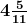cm. How many revolutions will it make in moving 6.3 km?

Solutions:-

From the question it is given that,

Diameter of a cycle wheel =cm = 49/11 cm

We know that, circumference of circle = πd

= 22/7 × 49/11

= 14 cm

= 1.4 m

Then, distance travelled = 6.3 km = 6,300 m

Therefore, number of revolutions = 6,300/0.14 = 45,000

13. The area of a circle ring enclosed between two concentric circles is 88 cm2. Find the radii of the two circles, if their difference is 1 cm.

Solution:-

Let us assume r be the radius of the inner circle,

And (r + 1) be the radius of the outer circle

Then,

Area of circular ring = Area of outer circle – area of inner circle

π(r + 1)2 – πr2 = 88

π(r2 + 2r + 1) – πr2 = 88

πr2 + 2πr + π – πr2 = 88

On simplification we get,

π(2r + 1) = 88

22/7(2r + 1) = 88

2r + 1 = 88 × 7/22

2r + 1 = 28

2r = 28 – 1

2r = 27

r = 27/2

r = 13.5 cm

(r + 1) = 13.5 + 1 = 14.5 cm

Therefore, radii of the two circles are 13.5 cm and 14.5 cm.

14. Find the area enclosed between two concentric circles, if their radii are 6 cm and 13 cm respectively.

Solution:-

From the question it is given that,

Radii of the two concentric circles are 6cm and 13 cm respectively.

r1 = 6 cm

r2 = 13 cm

We know that,

Area between two concentric circles = Areas of larger circle – area of smaller circle

Area of circle = πr2

= πr12 – πr22

= [(22/7) × 62] – [(22/7) – 132]

= 531.1429 – 113.1429

= 418 cm2

Therefore, area enclosed between two concentric circles is 418 cm2.

15. The area between the circumferences of two concentric circles is 2464 cm2. If the inner circle has circumference of 132 cm, calculate the radius of outer circle.

Solution:-

From the question it is given that,

The area between the circumferences of two concentric circles is 2464 cm2.

Circumference of inner circle = 132 cm

We know that, circumference = 2πr

132 = 2πr

r = 132/2π

r = (132 × 7)/(2 × 22)

r = 21 cm

So, radius of inner circle is 21 cm.

Then, area of inner circle = πr2

= 22/7 × 212

= 1,386 cm2

Area of outer circle = area of inner circle + area of concentric circles

= (1386 + 2464) cm2

= 3,850 cm2

Let R be the radius of outer circle,

Then, we know that area of outer circle = πR2

3,850 = πR2

R2 = (3,850 × 7)/22

R2 = 1225

R = √1225

R = 35 cm

Therefore, radius of outer circle is 35 cm.